In computational statistics, there are often several ways to solve the same problem. For example, there are many ways to solve for the least-squares solution of a linear regression model. A SAS programmer recently mentioned that some open-source software uses the QR algorithm to solve least-squares regression problems and asked how that compares with SAS. This article shows how to estimate a least-squares regression model by using the QR method in SAS. It shows how to use the QR method in an efficient way.

Solving the least-squares problem

Before discussing the QR method, let's briefly review other ways to construct a least-squares solution to a regression problem. In a regression problem, you have an n x m data matrix, X, and an n x 1 observed vector of responses, y. When n > m, this is an overdetermined system and typically there is no exact solution. Consequently, the goal is to find the m x 1 vector of regression coefficients, b, such that the predicted values (X b) are as close as possible to the observed values. If b is a least-squares solution, then b minimizes the vector norm || X b - y ||2, where ||v||2 is the sum of the squares of the components of a vector.

Using calculus, you can show that the solution vector, b, satisfies the normal equations (X`X) b = X`y. The normal equations have a unique solution when the crossproduct matrix X`X is nonsingular. Most numerical algorithms for least-squares regression start with the normal equations, which have nice numerical properties that can be exploited.

Creating a design matrix

The first step of solving a regression problem is to create the design matrix. For continuous explanatory variables, this is easy: You merely append a column of ones (the intercept column) to the matrix of the explanatory variables. For more complicated regression models that contain manufactured effects such as classification effects, interaction effects, or spline effects, you need to create a design matrix. The GLMSELECT procedure is the best way to create a design matrix for fixed effects in SAS. The following call to PROC GLMSELECT writes the design matrix to the DesignMat data set. The call to PROC REG estimates the regression coefficients:

 proc glmselect data=Sashelp.Class outdesign=DesignMat; /* write design matrix */ class Sex; model Weight = Height Sex Height*Sex/ selection=none; run; /* test: use the design matrix to estimate the regression coefficients */ proc reg data=DesignMat plots=none; model Weight = Height Sex_F Height_Sex_F; run;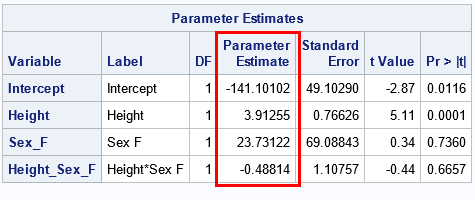The goal of the rest of this article is to reproduce the regression estimates by using various other linear algebra operations. For each method, we want to produce the same estimates: {-141.10, 3.91, 23.73, -0.49}.

Linear regression: A linear algebraic approach

I've previously discussed the fact that most SAS regression procedures use the sweep operator to construct least-squares solutions. Another alternative is to use the SOLVE function in SAS/IML:

 proc iml; use DesignMat; read all var {'Intercept' 'Height' 'Sex_F' 'Height_Sex_F'} into X; read all var {'Weight'} into Y; close;   /* form normal equations */ XpX = X`*X; Xpy = X`*y;   /* an efficient numerical solution: solve a particular RHS */ b = solve(XpX, Xpy); print b[L="SOLVE" F=D10.4];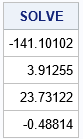The SOLVE function is very efficient and gives the same parameter estimates as the SWEEP operator (which was used by PROC REG). Using the SOLVE function on the system A*b=z is mathematically equivalent to using the matrix inverse to find b=A-1z. However, the INV function explicitly forms the inverse matrix, whereas the SOLVE function does not. Consequently, the SOLVE function is faster and more efficient than using the following SAS/IML statement:

 /* a less efficient numerical solution */ Ainv = inv(XpX); /* explicitly form the (m x m) inverse matrix */ b = Ainv*Xpy; /* apply the inverse matrix to RHS */ print b[L="INV" F=D10.4];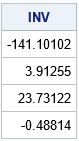For a performance comparison of the SOLVE and INV functions, see the article, "Solving linear systems: Which technique is fastest?"

An introduction to the QR method for solving linear equations

There are many ways to solve the normal equations. The SOLVE (and INV) functions use the LU decomposition. An alternative is the QR algorithm, which is slower but can be more accurate for ill-conditioned systems. You can apply the QR decomposition to the normal equations or to the original design matrix. This section discusses the QR decomposition of the design matrix. A subsequent article discusses decomposing the data matrix directly.

Let's see how the QR algorithm solves the normal equations. You can decompose the crossproduct matrix as the product of an orthogonal matrix, Q, and an upper-triangular matrix, R. If A = X`X, then A = QR. (I am ignoring column pivoting, which is briefly discussed below.) The beauty of an orthogonal matrix is that the transpose equals the inverse. This means that you can reduce the system A b = (X` y) to a triangular system R b = Q` (X` y), which is easily solved by using the TRISOLV function in SAS/IML.

The SAS/IML version of the QR algorithm is a version known as "QR with column pivoting." Using column pivoting improves solving rank-deficient systems and provides better numerical accuracy. However, when the algorithm uses column pivoting, you are actually solving the system AP = QR, where P is a permutation matrix. This potentially adds some complexity to dealing with the QR algorithm. Fortunately, the TRISOLVE function supports column pivoting. If you use the TRISOLV function, you do not need to worry about whether pivoting occurred or not.

Using the QR method in SAS/IML

Recall from the earlier example that it is more efficient to use the SOLVE function than the INV function. The reason is that the INV function explicitly constructs an m x m matrix, which then is multiplied with the right-hand side (RHS) vector to obtain the answer. In contrast, the SOLVE function never forms the inverse matrix. Instead, it directly applies transformations to the RHS vector.

The QR call in SAS/IML works similarly. If you do not supply a RHS vector, v, then the QR call returns the full m x m matrix, Q. You then must multiply Q` v yourself. If you do supply the RHS vector, then the QR call returns Q` v without ever forming Q. This second method is more efficient,

See the documentation of the QR call for the complete syntax. The following call uses the inefficient method in which the Q matrix is explicitly constructed:

 /* It is inefficient to factor A=QR and explicitly solve the equation R*b = Q`*c */ call QR(Q, R, piv, lindep, XpX); /* explicitly form the (m x m) matrix Q */ c = Q`*Xpy; /* apply the inverse Q matrix to RHS */ b = trisolv(1, R, c, piv); /* equivalent to b = inv(R)*Q`*c */ print b[L="General QR" F=D10.4];

In contrast, the next call is more efficient because it never explicitly forms the Q matrix:

 /* It is more efficient to solve for a specific RHS */ call QR(c, R, piv, lindep, XpX, ,Xpy); /* returns c = Q`*Xpy */ b = trisolv(1, R, c, piv); /* equivalent to b = inv(R)*Q`*c */ print b[L="Specific QR" F=D10.4];

The output is not shown, but both calls produce estimates for the regression coefficients that are exactly the same as for the earlier examples. Notice that the TRISOLV function takes the pivot vector (which represents a permutation matrix) as the fourth argument. That means you do not need to worry about whether column pivoting occurred during the QR algorithm.

QR applied to the design matrix

As mentioned earlier, you can also apply the QR algorithm to the design matrix, X, and the QR algorithm will return the least-square solution without ever forming the normal equations. This is shown in a subsequent article, which also compares the speed of the various methods for solving the least-squares problem.

Summary

This article discusses three ways to solve a least-squares regression problem. All start by constructing the normal equations: (X`X) b = X` y. The solution of the normal equations (b) is the vector that minimizes the squared differences between the predicted values, X b, and the observed responses, y. This article discusses

• the SWEEP operator, which is used by many SAS regression procedures
• the SOLVE and INV function, which use the LU factorization
• the QR call, which implements the QR algorithm with column pivoting

A follow-up article compares the performance of these methods and of another QR algorithm, which does not use the normal equations.

The post The QR algorithm for least-squares regression appeared first on The DO Loop.In general, it is hard to simulate multivariate data that has a specified correlation structure. Copulas make that task easier for continuous distributions. A previous article presented the geometry behind a copula and explained copulas in an intuitive way. Although I strongly believe that statistical practitioners should be familiar with statistical theory, you do not need to be an expert in copulas to use them to simulate multivariate correlated data. In SAS, the COPULA procedure in SAS/ETS software provides a syntax for simulating data from copulas. This article shows how to use PROC COPULA to simulate data that has a specified rank correlation structure. (Similar syntax applies to the CCOPULA procedure in SAS Econometrics, which can perform simulations in parallel in SAS Viya.)

Example data

Let's choose some example data to use for the simulation study. Suppose you want to simulate data that have marginal distributions and correlations that are similar to the joint distribution of the MPG_City, Weight, and EngineSize variables in the Sashelp.Cars data set. For ease of discussion, I rename these variables X1, X2, and X3 in this article. The following call to PROC CORR visualizes the univariate and bivariate distributions for these data:

 /* for ease of discussion, rename vars to X1, X2, X3 */ data Have; set Sashelp.Cars(keep= MPG_City Weight EngineSize); label MPG_City= Weight= EngineSize=; rename MPG_City=X1 Weight=X2 EngineSize=X3; run;   /* graph original (renamed) data */ ods graphics / width=500px height=500px; proc corr data=Have Spearman noprob plots=matrix(hist); var X1 X2 X3; ods select SpearmanCorr MatrixPlot; run;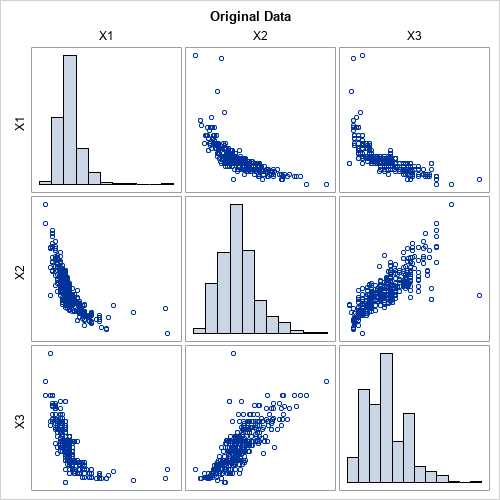The graph shows the marginal and bivariate distributions for these data. The goal of this article is to use PROC COPULA to simulate a random sample that looks similar to these data. The output from PROC CORR includes a table of Spearman correlations (not shown), which are Corr(X1,X2)=-0.865, Corr(X1,X3)=-0.862, and Corr(X2,X3)=0.835.

Using PROC COPULA to simulate data

As explained in my previous article about copulas, the process of simulating data involves several conceptual steps. For each step, PROC COPULA provides a statement or option that performs the step:

1. The multivariate distribution is defined by specifying a marginal distribution for each variable and a correlation structure for the joint distribution. In my previous article, these were created "out of thin air." However, in practice, you often want to simulate random samples that match the correlation and marginal distributions of real data. In PROC COPULA, you use the DATA= option and the VAR statement to specify real data.
2. Choose a copula family and use the data to estimate the parameters of the copula. I've only discussed the Gaussian copula. For the Gaussian copula, the sample covariance matrix estimates the parameters for the copula, although PROC COPULA uses maximum likelihood estimates instead of the sample covariance. You use the FIT statement to fit the parameters of the copula from the data.
3. Simulate from the copula. For the normal copula, this consists of generating multivariate normal data with the given rank correlations. These simulated data are transformed to uniformity by applying the normal CDF to each component. You use the SIMULATE statement to simulate the data. If you want to see the uniform marginals, you can use the OUTUNIFORM= option to store them to a SAS data set, or you can use the PLOTS=(DATATYPE=UNIFORM) option to display a scatter plot matrix of the uniform marginals.
4. Transform the uniform marginals into the specified distributions by applying the inverse CDF transformation for each component. In PROC COPULA, you use the MARGINALS=EMPIRICAL option on the SIMULATE statement to create the marginal distributions by using the empirical CDF of the variables. You can use the OUT= option to write the simulated values to a SAS data set. You can use the PLOTS=(DATATYPE=ORIGINAL) option to display a scatter plot matrix of the simulated data.

The following call to PROC COPULA carries out these steps:

 %let N = 428; /* sample size */ proc copula data=Have; var X1 X2 X3; /* original data vars */ fit normal; /* choose normal copula; estimate covariance by MLE */ simulate / seed=1234 ndraws=&N marginals=empirical /* transform from copula by using empirical CDF of data */ out=SimData /* contains the simulated data */ plots=(datatype=both); /* optional: scatter plots of copula and simulated data */ /* optional: use OUTUNIFORM= option to store the copula */ ods select SpearmanCorrelation MatrixPlotSOrig MatrixPlotSUnif; run;

The primary result of the PROC COPULA call is an output data set called SimData. The data set contains random simulated data. The Spearman correlation of the simulated data is close to the Spearman correlation of the original data. The marginal distributions of the simulated variables are similar to the marginal distributions of the original variables. The following graph shows the distributions of the simulated data. You can compare this graph to the graph of the original data.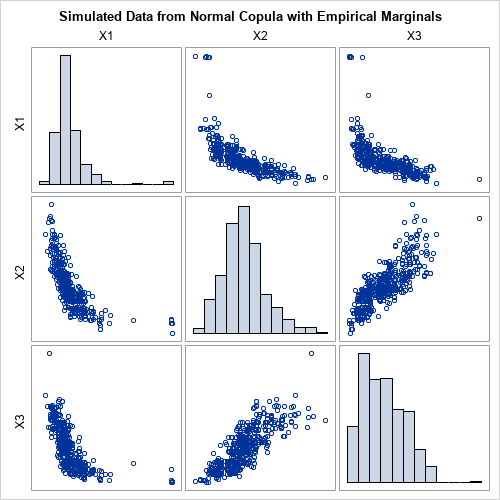When you use PROC COPULA, it is not necessary to visualize the copula, which encapsulates the correlation between the variables. However, if you want to visualize the copula, you can use the PLOTS= option to create a scatter plot matrix of the uniform marginals, as follows: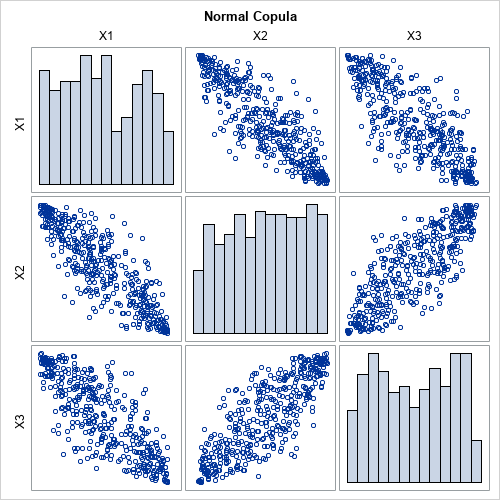Use PROC COPULA for Monte Carlo simulations

In Monte Carlo simulations, it is useful to have one SAS data set that contains B simulated samples. You can then use BY-group processing to analyze all simulated samples with a single call to a SAS procedure.

In PROC COPULA, the NDRAWS= option on the SIMULATE statement specifies how many observations to simulate. If your original data contains N observations, you can use NDRAWS=N. To get a total of B simulated samples, you can simulate N*B observations. You can then add an ID variable that identifies which observations belong to each sample, as follows:

 /* you can run a Monte Carlo simulation from the simulated data */ %let NumSamples = 1000; /* = B = number of Monte Carlo simulations */ %let NTotal = %eval(&N * &NumSamples); %put &=NTotal;   ods exclude all; proc copula data=Have; var X1 X2 X3; fit normal; simulate / seed=1234 ndraws=&NTotal marginals=empirical out=SimData; run; ods exclude none;   /* add ID variable that identifies each set of &N observations */ data MCData; set SimData; SampleID = ceil(_N_/&N); /* 1,1,...,1,2,2,....,2,3,3,... */ run;

You can use your favorite SAS procedure and the BY SAMPLEID statement to analyze each sample in the MCData data set.

Summary

Given a set of continuous variables, a copula enables you to simulate a random sample from a distribution that has the same rank correlation structure and marginal distributions as the specified variables. A previous article discusses the mathematics and the geometry of copulas. In SAS, the COPULA procedure enables you to simulate data from copulas. This article shows how to use PROC COPULA to simulate data. The procedure can create graphs that visualize the simulated data and the copula. The main output is a SAS data set that contains the simulated data.

The post Simulate multivariate correlated data by using PROC COPULA in SAS appeared first on The DO Loop.When I was a computer science student in the 1980s, our digital alphabet was simple and small. We could express ourselves with the letters A..Z (and lowercase a..z) and numbers (0..9) and a handful of punctuation and symbols. Thanks to the ASCII standard, we could represent any of these characters in a single byte (actually just 7 bits). This allowed for a generous 128 different characters, and we had character slots to spare. (Of course for non-English and especially non-latin characters we had to resort to different code pages...but that was before the Internet forced us to work together. Before Unicode, we lived in a digital Tower of Babel.)

Even with the limited character set, pictorial communication was possible with ASCII through the fun medium of "ASCII art." ASCII art is basically the stone-age version of emojis. For example, consider the shrug emoji: 🤷

Its ASCII-art ancestor is this: ¯\_(ツ)_/¯ While ASCII art currently enjoys a retro renaissance, the emoji has become indispensable in our daily communications.

Emojis before Unicode

Given the ubiquity of emojis in every communication channel, it's sometimes difficult to remember that just a few years ago emoji characters were devised and implemented in vendor-specific offerings. As the sole Android phone user in my house, I remember a time when my iPhone-happy family could express themselves in emojis that I couldn't read in the family group chat. Apple would release new emojis for their users, and then Android (Google) would leap frog with another set of their own fun symbols. But if you weren't trading messages with users of the same technology, then chunks of your text would be lost in translation.

Enter Unicode. A standard system for encoding characters that allows for multiple bytes of storage, Unicode has seemingly endless runway for adding new characters. More importantly, there is a standards body that sets revisions for Unicode characters periodically so everyone can use the same huge alphabet. In 2015, emoji characters were added into Unicode and have been revised steadily with universal agreement.

This standardization has helped to propel emojis as a main component of communication in every channel. Text messages, Twitter threads, Venmo payments, Facebook messages, Slack messages, GitHub comments -- everything accepts emojis. (Emojis are so ingrained and expected that if you send a Venmo payment without using an emoji and just use plain text, it could be interpreted as a slight or at the least as a miscue.)

For more background about emojis, read How Emjois Work (source: How Stuff Works).

Unicode is essential for emojis. In SAS, the use of Unicode is possible by way of UTF-8 encoding. If you work in a modern SAS environment with a diverse set of data, you should already be using ENCODING=UTF8 as your SAS session encoding. If you use SAS OnDemand for Academics (the free environment for any learner), this is already set for you. And SAS Viya offers only UTF-8 -- which makes sense, because it's the best for most data and it's how most apps work these days.

Emojis as data and processing in SAS

Emojis are everywhere, and their presence can enrich (and complicate) the way that we analyze text data. For example, emojis are often useful cues for sentiment (smiley face! laughing-with-tears face! grimace face! poop!). It's not unusual for a text message to be ALL emojis with no "traditional" words.

The website Unicode.org maintains the complete compendium of emojis as defined in the latest standards. They also provide the emoji definitions as data files, which we can easily read into SAS. This program reads all of the data as published and adds features for just the "basic" emojis:

 /* MUST be running with ENCODING=UTF8 */ filename raw temp; proc http url="https://unicode.org/Public/emoji/13.1/emoji-sequences.txt" out=raw; run;   ods escapechar='~'; data emojis (drop=line); length line \$ 1000 codepoint_range \$ 45 val_start 8 val_end 8 type \$ 30 comments \$ 65 saschar \$ 20 htmlchar \$ 25; infile raw ; input; line = _infile_; if substr(line,1,1)^='#' and line ^= ' ' then do; /* read the raw codepoint value - could be single, a range, or a combo of several */ codepoint_range = scan(line,1,';'); /* read the type field */ type = compress(scan(line,2,';')); /* text description of this emoji */ comments = scan(line,3,'#;');   /* for those emojis that have a range of values */ val_start = input(scan(codepoint_range,1,'. '), hex.); if find(codepoint_range,'..') > 0 then do; val_end = input(scan(codepoint_range,2,'.'), hex.); end; else val_end=val_start;   if type = "Basic_Emoji" then do; saschar = cat('~{Unicode ',scan(codepoint_range,1,' .'),'}'); htmlchar = cats('&#x',scan(codepoint_range,1,' .'),';'); end; output; end; run;   proc print data=emojis; run;

The "features" I added include the Unicode representation for an emoji character in SAS, which could then be used in any SAS report in ODS or any graphics produced in the SG procedures. I also added the HTML-encoded representation of the emoji, which uses the form &#xNNNN; where NNNN is the Unicode value for the character. Here's the raw data view: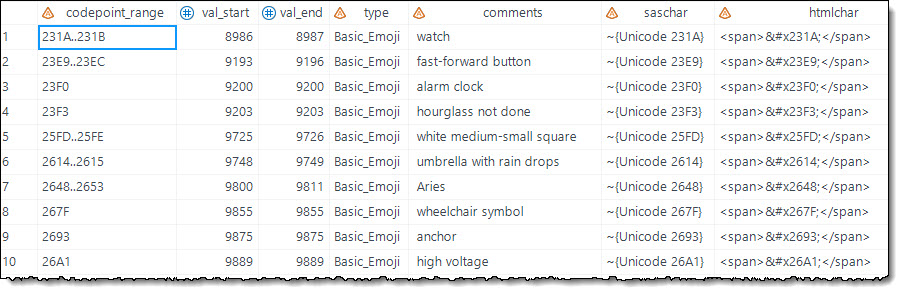When you PROC PRINT to an HTML destination, here's the view in the results browser: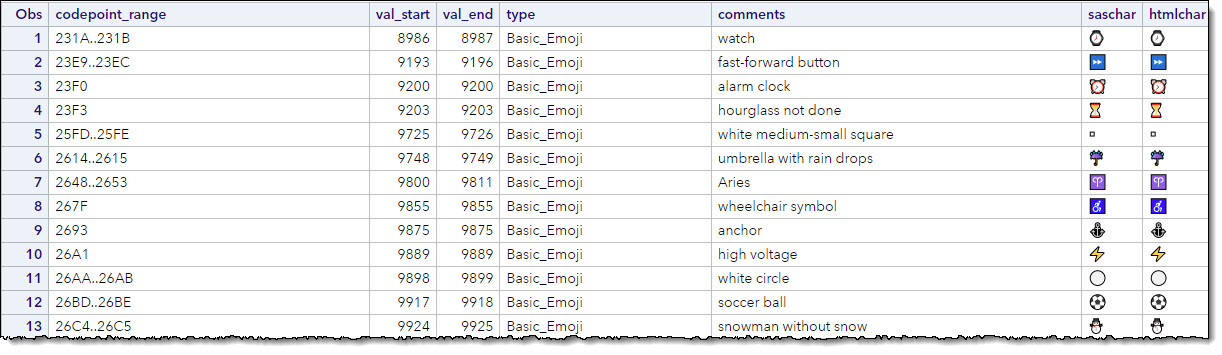In search of structured emoji data

The Unicode.org site can serve up the emoji definitions and codes, but this data isn't exactly ready for use within applications. One could work through the list of emojis (thousands of them!) and tag these with descriptive words and meanings. That could take a long time and to be honest, I'm not sure I could accurately interpret many of the emojis myself. So I began the hunt for data files that had this work already completed.

I found the GitHub/gemoji project, a Ruby-language code repository that contains a structured JSON file that describes a recent collection of emojis. From all of the files in the project, I need only one JSON file. Here's a SAS program that downloads the file with PROC HTTP and reads the data with the JSON libname engine:

 filename rawj temp; proc http url="https://raw.githubusercontent.com/github/gemoji/master/db/emoji.json" out=rawj; run;   libname emoji json fileref=rawj;

Upon reading these data, I quickly realized the JSON text contains the actual Unicode character for the emoji, and not the decimal or hex value that we might need for using it later in SAS.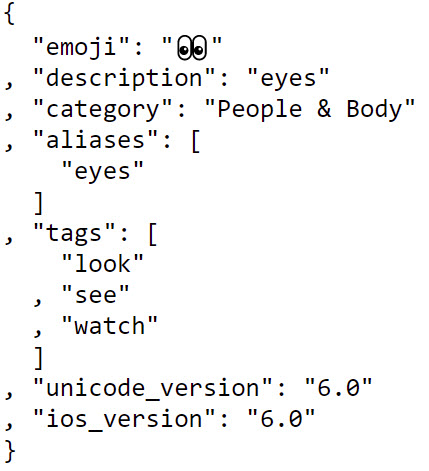I wanted to convert the emoji character to its numeric code. That's when I discovered the UNICODEC function, which can "decode" the Unicode sequence into its numeric values. (Note that some characters use more than one value in a sequence).

Here's my complete program, which includes some reworking of the tags and aliases attributes so I can have one record per emoji:

 filename rawj temp; proc http url="https://raw.githubusercontent.com/github/gemoji/master/db/emoji.json" out=rawj; run;   libname emoji json fileref=rawj;   /* reformat the tags and aliases data for inclusion in a single data set */ data tags; length ordinal_root 8 tags \$ 60; set emoji.tags; tags = catx(', ',of tags:); keep ordinal_root tags; run;   data aliases; length ordinal_root 8 aliases \$ 60; set emoji.aliases; aliases = catx(', ',of aliases:); keep ordinal_root aliases; run;   /* Join together in one record per emoji */ proc sql; create table full_emoji as select t1.emoji as emoji_char, unicodec(t1.emoji,'esc') as emoji_code, t1.description, t1.category, t1.unicode_version, case when t1.skin_tones = 1 then t1.skin_tones else 0 end as has_skin_tones, t2.tags, t3.aliases from emoji.root t1 left join tags t2 on (t1.ordinal_root = t2.ordinal_root) left join aliases t3 on (t1.ordinal_root = t3.ordinal_root) ; quit;   proc print data=full_emoji; run;

Here's a snippet of the report that includes some of the more interesting sequences: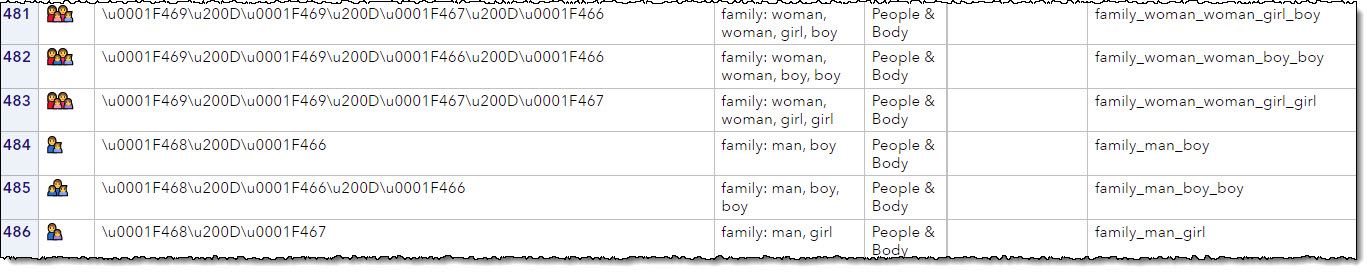The diversity and inclusion aspect of emoji glyphs is ever-expanding. For example, consider the emoji for "family":

• The basic family emoji code is \u0001F46A (👪)
• But since families come in all shapes and sizes, you can find a family that better represents you. For example, how about "family: man, man, girl, girl"? The code is \u0001F468\u200D\u0001F468\u200D\u0001F467\u200D\u0001F467, which includes the codes for each component "member" all smooshed together with a "zero-width joiner" (ZWJ) code in between (👨‍👨‍👧‍👧)
• All of the above, but with a dark-skin-tone modifier (\u0001F3FF) for 2 of the family members: \u0001F468\u0001F3FF\u200D\u0001F468\u200D\u0001F467\u200D\u0001F467\u0001F3FF (👨🏿‍👨‍👧‍👧🏿)

Conclusion: Emojis reflect society, and society adapts to emojis

As you might have noticed from that last sequence I shared, a single concept can call for many different emojis. As our society becomes more inclusive around gender, skin color, and differently capable people, emojis are keeping up. Everyone can express the concept in the way that is most meaningful for them. This is just one way that the language of emojis enriches our communication, and in turn our experience feeds back into the process and grows the emoji collection even more.

As emoji-rich data is used for reporting and for training of AI models, it's important for our understanding of emoji context and meaning to keep up with the times. Already we know that emoji use differs among different age generations and across other demographic groups. The use and application of emojis -- separate from the definition of emoji codes -- is yet another dimension to the data.

Our task as data scientists is to bring all of this intelligence and context into the process when we parse, interpret and build training data sets. The mechanics of parsing and producing emoji-rich data is just the start.

If you're encountering emojis in your data and considering them in your reporting and analytics, please let me know how! I'd love to hear from you in the comments.

The post How to work with emojis in SAS appeared first on The SAS Dummy.Having the ability to know what the best decision is in any given scenario sounds like a superpower. What may surprise you, is that this “superpower” already exists. SAS® calls it intelligent decisioning. Decisioning is a powerful tool in the business world. It is useful to both the company and [...]

3 ways industries are using AI-powered decisions was published on SAS Voices by Olivia OjedaIterative loops are one of the most powerful and imperative features of any programming language, allowing blocks of code to be automatically executed repeatedly with some variations. In SAS we call them DO-loops because they are defined by the iterative DO statements. These statements come in three distinct forms:

• DO with index variable
• DO UNTIL
• DO WHILE

In this blog post we will focus on the versatile iterative DO loops with index variable pertaining to SAS DATA steps, as opposed to its modest IML’s DO loops subset.

Iterative DO statement with index variable

The syntax of the DATA step’s iterative DO statement with index variable is remarkably simple yet powerful:

DO statement with index-variable

DO index-variable=specification-1 <, ...specification-n>;

...more SAS statements...

END;

It executes a block of code between the DO and END statements repeatedly, controlled by the value of an index variable. Given that angle brackets (< and >) denote “optional”, notice how index-variable requires at least one specification (specification-1) yet allows for multiple additional optional specifications (<, ...specification-n>) separated by commas.

Now, let’s look into the DO statement’s index-variable specifications.

Index-variable specification

Each specification denotes an expression, or a series of expressions as follows:

start-expression <TO stop-expression> <BY increment-expression> <WHILE (expression) | UNTIL (expression)>

Note that only start-expression is required here whereas <TO stop-expression>, <BY increment-expression>, and <WHILE (expression) or UNTIL (expression)> are optional.

Start-expression may be of either Numeric or Character type, while stop-expression and increment-expression may only be Numeric complementing Numeric start-expression.

Expressions in <WHILE (expression) | UNTIL (expression)> are Boolean Numeric expressions (numeric value other than 0 or missing is TRUE and a value of 0 or missing is FALSE).

Other iterative DO statements

For comparison, here is a brief description of the other two forms of iterative DO statement:

• The DO UNTIL statement executes statements in a DO loop repetitively until a condition is true, checking the condition after each iteration of the DO loop. In other words, if the condition is true at the end of the current loop it will not iterate anymore, and processing continues with the next statement after END. Otherwise, it will iterate.
• The DO WHILE statement executes statements in a DO loop repetitively while a condition is true, checking the condition before each iteration of the DO loop. That is if the condition is true at the beginning of the current loop it will iterate, otherwise it will not, and processing continues with the next statement after the END.

Looping over a list of index variable values/expressions

DO loops can iterate over a list of index variable values. For example, the following DO-loop will iterate its index variable values over a list of 7, 13, 5, 1 in the order they are specified:

 data A; do i=7, 13, 5, 1; put i=; output; end; run;

This is not yet another form of iterative DO loop as it is fully covered by the iterative DO statement with index variable definition. In this case, the first value (7) is the required start expression of the required first specification, and all subsequent values (13, 5 and 1) are required start expressions of the additional optional specifications.

Similarly, the following example illustrates looping over a list of index variable character values:

 data A1; length j \$4; do j='a', 'bcd', 'efgh', 'xyz'; put j=; output; end; run;

Since DO loop specifications denote expressions (values are just instances or subsets of expressions), we can expand our example to a list of actual expressions:

 data B; p = constant('pi'); do i=round(sin(p)), sin(p/2), sin(p/3); put i=; output; end; run;

In this code DO-loop will iterate its index variable over a list of values defined by the following expressions: round(sin(p)), sin(p/2), sin(p/3).

Infinite loops

Since <TO stop> is optional for the index-variable specification, the following code is perfectly syntactically correct:

 data C; do j=1 by 1; output; end; run;

It will result in an infinite (endless) loop in which resulting data set will be growing indefinitely.

While unintentional infinite looping is considered to be a bug and programmers’ anathema, sometimes it may be used intentionally. For example, to find out what happens when data set size reaches the disk space capacity… Or instead of supplying a “big enough” hard-coded number (which is not a good programming practice) for the loop’s TO expression, we may want to define an infinite DO-loop and take care of its termination and exit inside the loop. For example, you can use IF exit-condition THEN LEAVE; or IF exit-condition THEN STOP; construct.

LEAVE statement immediately stops processing the current DO-loop and resumes with the next statement after its END.

STOP statement immediately stops execution of the current DATA step and SAS resumes processing statements after the end of the current DATA step.

The exit-condition may be unrelated to the index-variable and be based on some events occurrence. For instance, the following code will continue running syntactically “infinite” loop, but the IF-THEN-LEAVE statement will limit it to 200 seconds:

 data D; start = datetime(); do k=1 by 1; if datetime()-start gt 200 then leave; /* ... some processing ...*/ output; end; run;

You can also create endless loop using DO UNTIL(0); or DO WHILE(1); statement, but again you would need to take care of its termination inside the loop.

Changing “TO stop” within DO-loop will not affect the number of iterations

If you think you can break out of your DO loop prematurely by adjusting TO stop expression value from within the loop, you may want to run the following code snippet to prove to yourself it’s not going to happen:

 data E; n = 4; do i=1 to n; if i eq 2 then n = 2; put i=; output; end; run;

This code will execute DO-loop 4 times despite that you change value of n from 4 to 2 within the loop.

According to the iterative DO statement documentation, any changes to stop made within the DO group do not affect the number of iterations. Instead, in order to stop iteration of DO-loop before index variable surpasses stop, change the value of index-variable so that it becomes equal to the value of stop, or use LEAVE statement to jump out of the loop. The following two examples will do just that:

 data F; do i=1 to 4; put i=; if i eq 2 then i = 4; output; end; run;   data G; do i=1 to 4; put i=; if i eq 2 then leave; output; end; run;

Know thy DO-loop specifications

Here is a little attention/comprehension test for you.

How many times will the following DO-loop iterate?

 data H; do i=1, 7, 3, 6, 2 until (i>3); put i=; output; end; run;

If your answer is 2, you need to re-read the whole post from the beginning (I am only partly joking here).

You may easily find out the correct answer by running this code snippet in SAS. If you are surprised by the result, just take a closer look at the DO statement: there are 5 specifications for the index variable here (separated by commas) whereas UNTIL (expression) belongs to the last specification where i=2. Thus, UNTIL only applies to a single value of i=2 (not to any previous specifications of i =1,7,3,6); therefore, it has no effect as it is evaluated at the end of each iteration.

Now consider the following DO-loop definition:

 data Z; pi = constant('pi'); do x=3 while(x>pi), 10 to 1 by -pi*3, 20, 30 to 35 until(pi); put x=; output; end; run;

I hope after reading this blog post you can easily identify the index variable list of values the DO-loop will iterate over. Feel free to share your solution and explanation in the comments section below.

Do you find this post useful? Do you have questions, other secrets, tips or tricks about the DO loop? Please share with us below.

Little known secrets of DO-loops with index variables was published on SAS Users.Do you know what a copula is? It is a popular way to simulate multivariate correlated data. The literature for copulas is mathematically formidable, but this article provides an intuitive introduction to copulas by describing the geometry of the transformations that are involved in the simulation process. Although there are several families of copulas, this article focuses on the Gaussian copula, which is the simplest to understand.

This article shows the geometry of copulas. This article and its example are based on Chapter 9 of Simulating Data with SAS (Wicklin, 2013). A previous article shows the geometry of the Iman-Conover transformation, which is an alternative way to create simulated data that have a specified rank correlation structure and specified marginal distributions.

Simulate data by using a copula

Recall that you can use the CDF function to transform any distribution to the uniform distribution. Similarly, you can use the inverse CDF to transform the uniform distribution to any distribution. To simulate correlated multivariate data from a Gaussian copula, follow these three steps:

1. Simulate correlated multivariate normal data from a correlation matrix. The marginal distributions are all standard normal.
2. Use the standard normal CDF to transform the normal marginals to the uniform distribution.
3. Use inverse CDFs to transform the uniform marginals to whatever distributions you want.

The transformation in the second and third steps are performed on the individual columns of a data matrix. The transformations are monotonic, which means that they do not change the rank correlation between the columns. Thus, the final data has the same rank correlation as the multivariate normal data in the first step.

The next sections explore a two-dimensional example of using a Gaussian copula to simulate correlated data where one variable is Gamma-distributed and the other is Lognormally distributed. The article then provides intuition about what a copula is and how to visualize it. You can download the complete SAS program that generates the example and creates the graphs in this article.

A motivating example

Suppose that you want to simulate data from a bivariate distribution that has the following properties:

• The rank correlation between the variables is approximately 0.6.
• The marginal distribution of the first variable, X1, is Gamma(4) with unit scale.
• The marginal distribution of the second variable, X2, is lognormal with parameters μ=0.5 and σ=0.8. That is, log(X2) ~ N(μ, σ).

The hard part of a multivariate simulation is getting the correlation structure correct, so let's start there. It seems daunting to generate a "Gamma-Lognormal distribution" with a correlation of 0.6, but it is straightforward to generate a bivariate NORMAL distribution with that correlation. Let's do that. Then we'll use a series of transformations to transform the normal marginal variables into the distributions that we want while preserving the rank correlation at each step.

Simulate multivariate normal data

The SAS/IML language supports the RANDNORMAL function, which can generate multivariate normal samples, as shown in the following statements:

 proc iml; N = 1e4; call randseed(12345); /* 1. Z ~ MVN(0, Sigma) */ Sigma = {1.0 0.6, 0.6 1.0}; Z = RandNormal(N, {0,0}, Sigma); /* Z ~ MVN(0, Sigma) */

The matrix Z contains 10,000 observations drawn from a bivariate normal distribution with correlation coefficient ρ=0.6. Because the mean vector is (0,0) and the covariance parameter is a correlation matrix, the marginal distributions are standard normal. The following graph shows a scatter plot of the bivariate normal data along with histograms for each marginal distribution.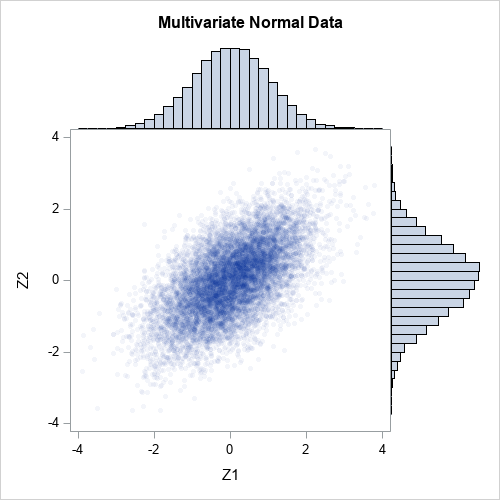Transform marginal distributions to uniform

The first step is to transform the normal marginals into a uniform distribution by using the probability integral transform (also known as the CDF transformation). The columns of Z are standard normal, so Φ(X) ~ U(0,1), where Φ is the cumulative distribution function (CDF) for the univariate normal distribution. In SAS/IML, the CDF function applies the cumulative distribution function to all elements of a matrix, so the transformation of the columns of Z is a one-liner:

 /* 2. transform marginal variables to U(0,1) */ U = cdf("Normal", Z); /* U_i are correlated U(0,1) variates */

The columns of U are samples from a standard uniform distribution. However, the columns are not independent. Because the normal CDF is a monotonic transformation, it does not change the rank correlation between the columns. That is, the rank correlation of U is the same as the rank correlation of Z:

 /* the rank correlations for Z and U are exactly the same */ rankCorrZ = corr(Z, "Spearman"); rankCorrU = corr(U, "Spearman"); print rankCorrZ rankCorrU;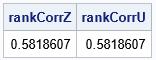The following graph shows a scatter plot of the transformed data along with histograms for each marginal distribution. The histograms show that the columns U1 and U2 are uniformly distributed on [0,1]. However, the joint distribution is correlated, as shown in the following scatter plot: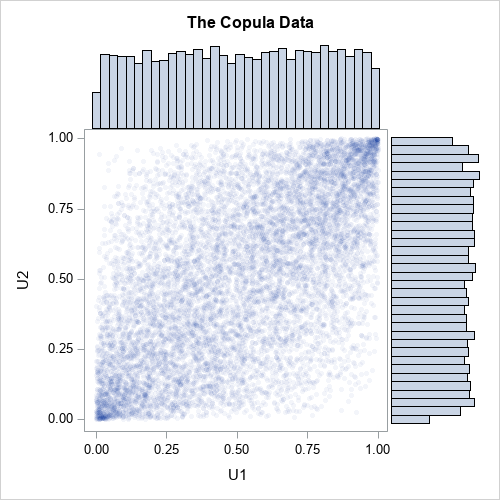Transform marginal distributions to any distribution

Now comes the magic. One-dimensional uniform variates are useful because you can transform them into any distribution! How? Just apply the inverse cumulative distribution function of whatever distribution you want. For example, you can obtain gamma variates from the first column of U by applying the inverse gamma CDF. Similarly, you can obtain lognormal variates from the second column of U by applying the inverse lognormal CDF. In SAS, the QUANTILE function applies the inverse CDF, as follows:

 /* 3. construct the marginals however you wish */ gamma = quantile("Gamma", U[,1], 4); /* gamma ~ Gamma(alpha=4) */ LN = quantile("LogNormal", U[,2], 0.5, 0.8); /* LN ~ LogNormal(0.5, 0.8) */ X = gamma || LN;   /* check that the rank correlation still has not changed */ rankCorrX = corr(X, "Spearman"); print rankCorrZ rankCorrX;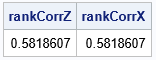The first column of X is gamma-distributed. The second column of X is lognormally distributed. But because the inverse of a continuous CDF is monotonic, the column transformations do not change the rank correlation between the columns. The following graph shows a scatter plot of the newly transformed data along with histograms for each marginal distribution. The histograms show that the columns X1 and X2 are distributed as gamma and lognormal, respectively. The joint distribution is correlated.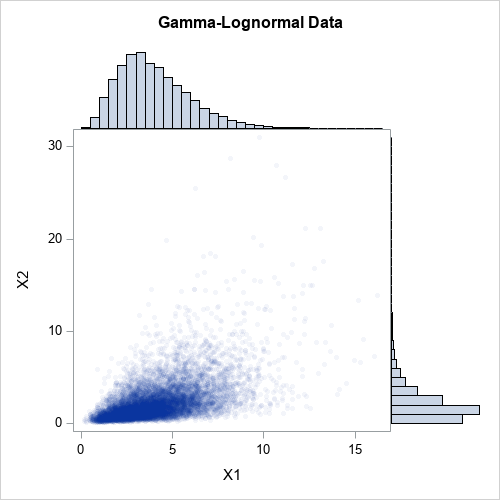For multivariate normal data, the Pearson correlation is close to the rank correlation. However, that is not true for nonnormal distributions. The CDF and inverse-CDF transformations are nonlinear, so the Pearson correlation is not preserved when you transform the marginal. For example, the following statements compute the Pearson correlation for Z (the multivariate normal data) and for X (the gamma-lognormal data):

 rhoZ = corr(Z, "Pearson"); rhoX = corr(X, "Pearson"); print rhoZ rhoX;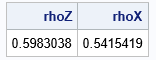Chapter 9 of Wicklin (2013), discusses the fact that you can adjust the correlation for Z and it will affect the correlation for X in a complicated manner. With a little work, you can choose a correlation for Z that will result in a specified correlation for X. For example, if you want the final Pearson correlation for X to be 0.6, you can use 0.642 as the correlation for Z:

 /* re-run the example with new correlation 0.642 */ Sigma = {1.0 0.642, 0.642 1.0}; newZ = RandNormal(N, {0,0}, Sigma); newU = cdf("Normal", newZ); /* columns of U are U(0,1) variates */ gamma = quantile("Gamma", newU[,1], 4); /* gamma ~ Gamma(alpha=4) */ expo = quantile("Expo", newU[,2]); /* expo ~ Exp(1) */ newX = gamma || expo;   rhoZ = corr(newZ, "Pearson"); rhoX = corr(newX, "Pearson"); print rhoZ rhoX;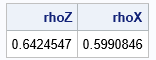Success! The Pearson correlation between the gamma and lognormal variables is 0.6. In general, this is a complicated process because the value of the initial correlation depends on the target value and on the specific forms of the marginal distributions. Finding the initial value requires finding the root of an equation that involves the CDF and inverse CDF.

Higher dimensional data

This example in this article generalizes to higher-dimensional data in a natural way. The SAS program that generates the example in this article also includes a four-dimensional example of simulating correlated data. The program simulates four correlated variables whose marginal distributions are distributed as gamma, lognormal, exponential, and inverse Gaussian distributions. The following panel shows the bivariate scatter plots and marginal histograms for this four-dimensional simulated data.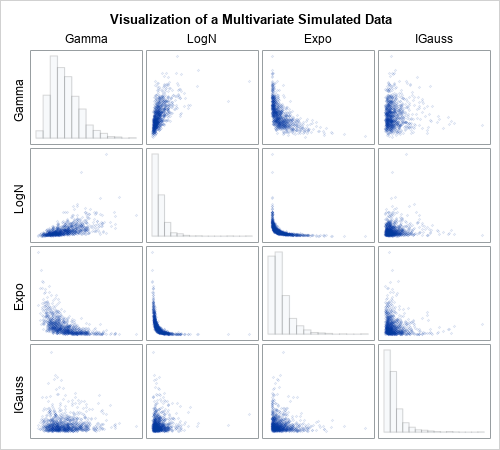What is a copula?

I've shown many graphs, but what is a copula? The word copula comes from a Latin word (copulare) which means to bind, link, or join. The same Latin root gives us the word "copulate." A mathematical copula is a joint probability distribution that induces a specified correlation structure among independent marginal distributions. Thus, a copula links or joins individual univariate distributions into a joint multivariate distribution that has a specified correlation structure.

Mathematically, a copula is any multivariate cumulative distribution function for which each component variable has a uniform marginal distribution on the interval [0, 1]. That's it. A copula is a cumulative distribution function whose domain is the cube [0,1]d. So the second graph in this article is the graph most nearly related to the copula for the bivariate data, since it shows the relationship between the uniform marginals.

The scatter plot shows the density, not the cumulative distribution. However, you can use the data to estimate the bivariate CDF. The following heat map visualizes the Gaussian copula for the correlation of 0.6: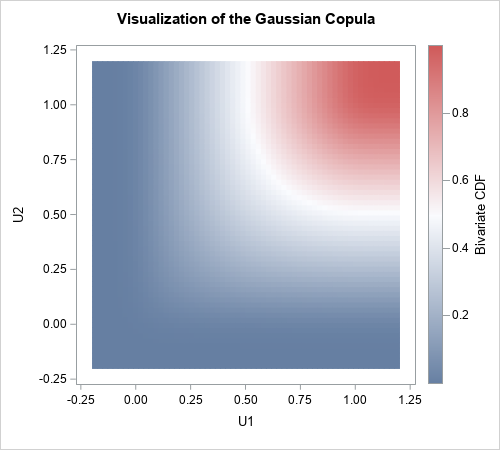Notice that this function does not depend on the final marginal distributions. It depends only on the multivariate normal distribution and the CDF transformation that produces the uniform marginals. In that sense, the copula captures only the correlation structure, without regard for the final form of the marginal distributions. You can use this same copula for ANY set of marginal distributions because the copula captures the correlation structure independently of the final transformations of the marginals.

Why are copulas important?

A remarkable theorem (Sklar’s theorem) says that every joint distribution can be written as a copula and a set of marginal distributions. If the marginals are continuous, then the copula is unique. Let that sink in for a second: Every continuous multivariate probability distribution can be expressed in terms of its marginal distributions and a copula. Every one. So, if you can understand copulas you can understand all multivariate distributions. Powerful math, indeed!

For simulation studies, you can use the converse of Sklar's theorem, which is also true. Specifically, if you have a set of d uniform random variables and a set of marginal distribution functions, a copula transforms the d components into a d-dimensional probability distribution.

Summary

Copulas are mathematically sophisticated. However, you can use copulas to simulate data without needing to understand all the mathematical details. This article presents an example of using a Gaussian copula to simulate multivariate correlated data. It shows the geometry at each step of the three-step process:

1. Simulate data from a multivariate normal distribution with a known correlation matrix.
2. Use the normal CDF to transform the marginal distributions to uniform.
3. Use inverse CDFs to obtain any marginal distributions that you want.

The result is simulated correlated multivariate data that has the same rank correlation as the original simulated data but has arbitrary marginal distributions.

This article uses SAS/IML to show the geometry of the copula transformations. However, SAS/ETS software provides PROC COPULA, which enables you to perform copula modeling and simulation in a single procedure. A subsequent article shows how to use PROC COPULA.

The post An introduction to simulating correlated data by using copulas appeared first on The DO Loop.To successfully adapt and reinvent business decisions amid the uncertainty of the past year, organizations have leaned on support from their partner ecosystems. Together, SAS and its SAS Partner community have collaborated to find new answers to customers' toughest challenges and to drive innovation and digital transformation. In honor of this, [...]

Awarding excellence in innovation was published on SAS Voices by Kate UlvelingHippocrates once said, “Let food be thy medicine.” For food vendors, this advice could be communicated another way: Stock more fresh fruit, protein and vegetables. The periphery of the grocery store has led in the expansion of revenues – namely within produce, but also spanning seafood, bakery products and ready-to-eat [...]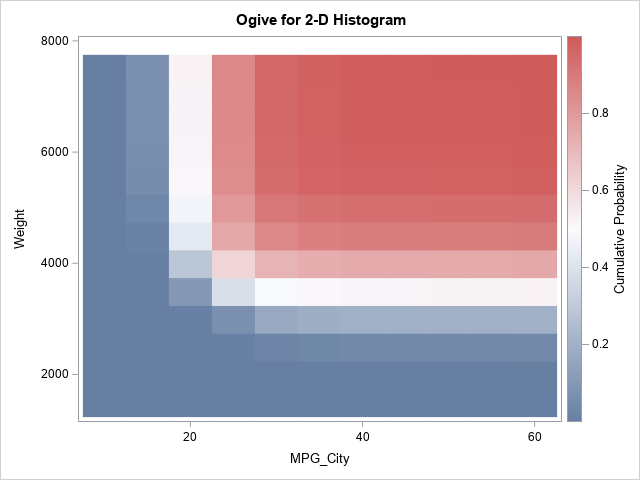A recent article about how to estimate a two-dimensional distribution function in SAS inspired me to think about a related computation: a 2-D cumulative sum. Suppose you have numbers in a matrix, X. A 2-D cumulative sum is a second matrix, C, such that the C[p,q] gives the sum of the cells of X for rows less than p and columns less than q. In symbols, C[p,q] = sum( X[i,j], i ≤ p, j ≤ q ).

This article shows a clever way to compute a 2-D cumulative sum and shows how to use this computation to compute a 2-D ogive, which is a histogram-based estimate of a 2-D cumulative distribution (CDF). An example of a 2-D ogive is shown to the right. You can compare the ogive to the 2-D CDF estimate from the previous article.

2-D cumulative sums

A naive computation of the 2-D cumulative sums would use nested loops to sum submatrices of X. If X is an N x M matrix, you might think that the cumulative sum requires computing N*M calls to the SUM function. However, there is a simpler computation that requires only N+M calls to the CUSUM function. You can prove by induction that the following algorithm gives the cumulative sums:

1. Compute the cumulative sums for each row. In a matrix language such as SAS/IML, this requires N calls to the CUSUM function.
2. Use the results of the first step and compute the "cumulative sums of the cumulative sums" down the columns. This requires M calls to the CUSUM function.

Thus, in the SAS/IML language, a 2-D cumulative sum algorithm looks like this:

 proc iml; /* compute the 2-D CUSUM */ start Cusum2D(X); C = j(nrow(X), ncol(X), .); /* allocate result matrix */ do i = 1 to nrow(X); C[i,] = cusum( X[i,] ); /* cusum across each row */ end; do j = 1 to ncol(X); C[,j] = cusum( C[,j] ); /* cusum down columns */ end; return C; finish; store module=(Cusum2D);   Count = { 7 6 1 3, 6 3 5 2, 1 2 4 1}; Cu = Cusum2D(Count); print Count, Cu;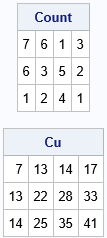The output shows the original matrix and the 2-D cumulative sums. For example, in the CU matrix, the (2,3) cell contains the sum of the submatrix Count[1:2, 1:3]. That is, the value 28 in the CU matrix is the sum (7+6+1+6+3+5) of values in the Count matrix.

From cumulative sums to ogives

Recall that an ogive is a cumulative histogram. If you start with a histogram that shows the number of observations (counts) in each bin, then an ogive displays the cumulative counts. If you scale the histogram so that it estimates the probability density, then the ogive estimates the univariate CDF.

A previous article showed how to construct an ogive in one dimension from the histogram. To construct a two-dimensional ogive, do the following:

• You start with a 2-D histogram. For definiteness, let's assume that the histogram displays the counts in each two-dimensional bin.
• You compute the 2-D cumulative sums, as shown in the previous section. If you divide the cumulative sums by the total number of observations, you obtain the cumulative proportions.
• The matrix of cumulative proportions is the 2-D ogive. However, by convention, an ogive starts at 0. Consequently, you should append a row and column of zeros to the left and top of the matrix of bin counts.

You can download the complete SAS/IML program that computes the ogive. The program defines two SAS/IML functions. The Hist2D function creates a matrix of counts for bivariate data. The Ogive2D function takes the matrix of counts and converts it to a matrix of cumulative proportions by calling the Cusum2D function in the previous section. It also pads the cumulative proportions with a row and a column of zeros. The function returns a SAS/IML list that contains the ogive and the vectors of endpoints for the ogive. The following statements construct an ogive from the bivariate data for the MPG_City and Weight variables in the Sashelp.Cars data set:

 proc iml; /* load computational modules */ load module=(Hist2D Cusum2D Ogive2D);   /* read bivariate data */ use Sashelp.Cars; read all var {MPG_City Weight} into Z; close;   * define cut points in X direction (or use the GSCALE function); cutX = do(10, 60, 5); cutY = do(1500, 7500, 500); Count = Hist2D(Z, cutX, cutY); /* matrix of counts in each 2-D bin */ L = Ogive2D(Count, cutX, cutY); /* return value is a list */ ogive = L\$'Ogive'; /* matrix of cumulative proportions */ print ogive[F=Best5. r=(L\$'YPts') c=(L\$'XPts')];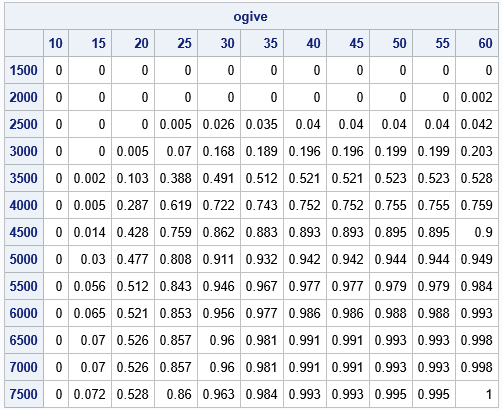The table shows the values of the ogive. You could use bilinear interpolation if you want to estimate the curve that corresponds to a specific probability, such as 0.5. You can also visualize the ogive by using a heat map, as shown at the top of this article. Notice the difference between the tabular and graphical views: rows increase DOWN in a matrix, but the Y-axis points UP in a graph. To compare the table and graph, you can reverse the rows of the table.

Summary

This article shows how to compute a 2-D cumulative sum. You can use the 2-D cumulative sum to create a 2-D ogive from the counts of a 2-D histogram. The ogive is a histogram-based estimate of the 2-D CDF. You can download the complete SAS program that computes 2-D cumulative sums, histograms, and ogives.

The post Compute 2-D cumulative sums and ogives appeared first on The DO Loop.